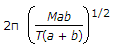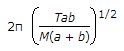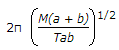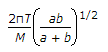# Civil Engineering - UPSC Civil Service Exam Questions

11.

The moment of inertia of a square cross-section of side 'b' about its diagonal is

 A. b4/3 B. b4/6 C. b4/8 D. b4/12

Explanation:

No answer description available for this question. Let us discuss.

12.

A particle of mass M is attached to a light horizontal wire which is stretched tightly between two fixed ends with a tension T. If a and b are the distances of the particle from the two ends, then the period of a small transverse oscillation of the particle is given by

 A.B.C.D.Explanation:

No answer description available for this question. Let us discuss.

13.

What is the ratio of the permissible bearing stress in power driven shop rivets relative to the yield stress of mild steel ?

 A. 1 B. 0.8 C. 0.6 D. 0.4

Explanation:

No answer description available for this question. Let us discuss.

14.

If the methyl orange alkalinity of water equals or exceeds total hardness, all of the harness is

 A. non-carbonate hardness B. carbonate hardness C. pseudo hardness D. negative non-carbonate hardness

Explanation:

No answer description available for this question. Let us discuss.

15.

Which one of the following statements is correct ? The number of unknowns to be determined in the stiffness method is equal to :

 A. the static indeterminacy B. the kinematic indeterminacy C. the sum of kinematic indeterminacy and static indeterminacy D. two times the number of supports